Tamilnadu Samacheer Kalvi 9th Maths Solutions Chapter 3 Algebra Ex 3.14

Solve by any one of the methods
Question 1.
The sum of a two digit number and the number formed by interchanging the digits is 110. If 10 is subtracted from the first number, the new number is 4 more than 5 times the sums of the digits of the first number. Find the first number.
Solution:
Let the two digit number be x y
Its place value = 10x + y
After interchanging the digits the number will be y x
Its place value = 10y + x
Their sum = 10x + y + 10y + x = 110
11x + 11y = 110
x + y = 10 ………….. (1)
If 10 is subtracted from the first number, the new number is 10x + y – 10
The sums of the digits of the first number is x + y
Its 4 more than 5 times is = 5(x + y) + 4
∴ 10x + y – 10 = 5x + 5y + 4
10x + y – 5x – 5y = 4 + 10
5x – 4y = 14 ………. (2)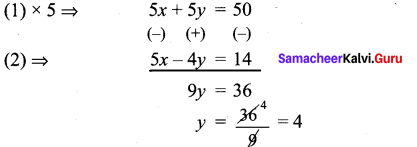Substitute y = 4 in (1)
x + 4 = 10
x = 10 – 4
x = 6
The first number is 64

Question 2.
The sum of the numerator and denominator of a fraction is 12. If the denominator is increased by 3, the fraction becomes $$\frac{1}{2}$$. Find the fraction.
Solution:
Let the numerator be x
Denominator be y
x + y = 12 ………. (1)
$$\frac{x}{y+3}=\frac{1}{2}$$
2x = y + 3
2x – y = 3 …………. (2)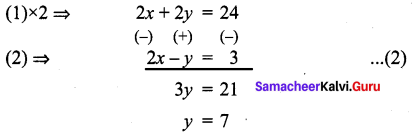Substitute y = 7 in (1)
x + 7 = 12
x = 12 – 7
x = 5
∴ The fraction is $$\frac{x}{y}=\frac{5}{7}$$

Question 3.
ABCD is a cyclic quadrilateral such that ∠A = (4y + 20)°, ∠B = (3y – 5)°, ∠C =(4x)° and ∠D = (7x + 5)°. Find the four angles.
Solution:
In a cyclic quadrilateral the sum of the four angles is 360° and
the sum of the opposite angles is 180°.
∠A + ∠C = 180°
4y + 20 + 4x = 180°
4x + 4y = 180 – 20
x + y = $$\frac{160}{4}$$
x + y = 40 ………….. (1)
∠B + ∠D = 180°
3y – 5 + 7x + 5 = 180
7x + 3y = 180 …………. (2)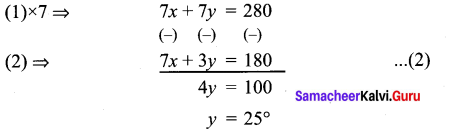Substitute y = 25° in (1)
x + 25 = 40
x = 40 – 25
x = 15°
∠A = (4y + 20)° = (4 × 25 + 20) = 100 + 20 = 120°
∠B = (3y – 5)° = (3 × 25° – 5) = 75° – 5° = 70°
∠C = (4x)° = 4 × 15° = 60°
∠D = (7x + 5)° = (7 × 15 + 5) = 105° + 5° = 110°
∴ ∠A = 120°, ∠B = 70°, ∠C = 60°, ∠D = 110°Question 4.
On selling a T.V. at 5% gain and a fridge at 10% gain, a shopkeeper gains ₹ 2000. But if he sells the T.V. at 10% gain and the fridge at 5% loss, he gains ₹ 1500 on the transaction. Find the actual price of the T.V. and the fridge.
Solution:
Let the actual price of a T.V. = x
Let the actual price of a Fridge = y
$$\frac{5}{100}$$x + $$\frac{10}{100}$$y = 2000
$$\frac{5}{100}$$ (x + 2y) = 2000
x + 2y = 2000 × $$\frac{100}{5}$$
x + 2y = 40000 ………. (1)
$$\frac{10}{100}$$ x – $$\frac{5}{100}$$y = 1500
2x – y = 1500 × $$\frac{100}{5}$$
2x – y = 30000 ……….. (2)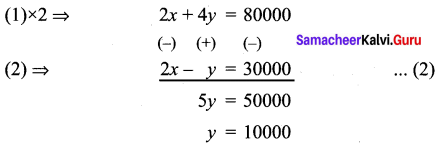Substitute y = 10000 in (1)
x + 2(10000) = 40000
x + 20000 = 40000
x = 40000 – 20000
x = 20000
∴ Actual price of T.V. = ₹ 20000
Actual price of Fridge = ₹ 10000

Question 5.
Two numbers are in the ratio 5 : 6. If 8 is subtracted from each of the numbers, the ratio becomes 4 : 5. Find the numbers.
Solution:
Let the two numbers be x, y
$$\frac{x}{y}=\frac{5}{6}$$
⇒ 6x = 5y
6x – 5y = 0 ………… (1)
$$\frac{x-8}{y-8}=\frac{4}{5}$$
⇒ 5(x – 8) = 4(y – 8)
5x – 40 = 4y – 32
5x – 4y = 40 – 32
5x – 4y =8 ………….. (2)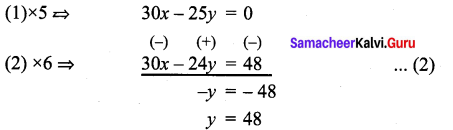Substitute y = 48 in (1)
6x – 5(48) = 0
6x – 240 = 0
6x = 240
x = $$\frac{240}{6}$$ = 40
$$\frac{x}{y}=\frac{40}{48}=\frac{5}{6}$$
∴ The numbers are in the ratio 5 : 6

Question 6.
4 Indians and 4 Chinese can do a piece of work in 3 days. While 2 Indians and 5 Chinese can finish it in 4 days. How long would it take for 1 Indian to do it? How long would it take for 1 Chinese to do it?
Solution:
Let for one Indian the rate of working be $$\frac{1}{x}$$
Let for one Chinese the rate of working be $$\frac{1}{y}$$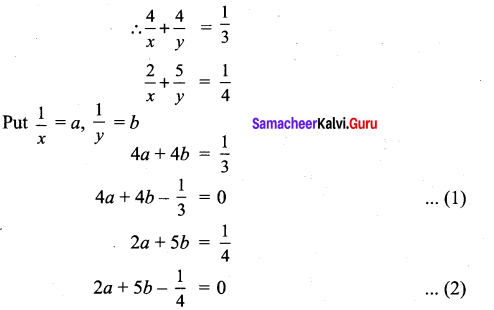For cross multiplication method, we write the co-efficients as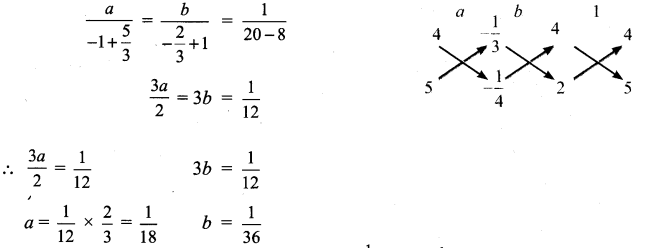∴ 1 Chinese can do the same piece of work = $$\frac{1}{y}$$ = b = $$\frac{1}{36}$$ = 36 days
1 Indian can do the piece of work = $$\frac{1}{x}$$ = a = $$\frac{1}{18}$$ = 18 days